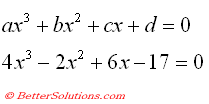# Cubic Equation

Lets consider the following cubic equation that we want to find a solution for.
One way to find a solution is to use the Solver add-in.
This add-in allows you to use a formula and specify a result in order to determine the necessary argument values.Solver uses a series of approximations to obtain a solution so it is not suprising that the final result will depend on the initial value.
Using constraints to pin point the exact roots